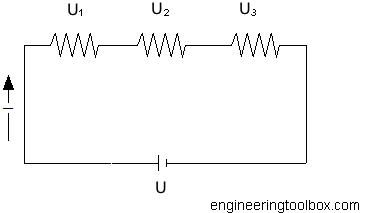Engineering ToolBox - Resources, Tools and Basic Information for Engineering and Design of Technical Applications!

# Electrical Series Circuits

## Voltage and current in electrical series circuits.

A series circuit has only one path for the electrons to flow.

The Series Circuit Rules:

1. The total current in the circuit is equal to the current in any other part of the circuit.
2. The total voltage in a series circuit is equal to the sum of the voltages across all parts in the circuit.
3. The total resistance in a series circuit is equal to the resistances of all parts in the circuit.In series circuits the sum of voltages is equal to the applied voltage and can be expressed as

U = U1 + U2 + ... + Un                  (1)

where

U = applied voltage from the battery or source (volts, V)

U1..n = voltage over each resistor (volts, V)

From Ohms Law

Un = In Rn                     (2)

where

In = current (amps, A)

Rn = resistance (ohms, Ω)

According rule 1 the currents in a series circuit are equal. This can be expressed as

I1 = I2 = .. = In                    (3)

- equation (1) can be modified to

R = R1 + R2 + .. + Rn                 (4)

where

R = total circuit resistance in the circuit (ohms, Ω)

### Example - Series Circuit

Three resistors - 1.25 Ω, 0.5 Ω and 1.5 Ω - are connected in series to a 12 V car battery. The total resistance in the circuit can be calculated as

R = (1.0 Ω) + (0.5 Ω) + (1.5 Ω)

= 3 Ω

The current in the series circuit can be calculated as

I = U / R

= 12 / 3

= 4 amps

The power dissipated in the circuit can be calculated

P = R I2

= (3 Ω) (4 amps)2

= 48 watts

## Related Topics

• ### Electrical

Electrical units, amps and electrical wiring, wire gauge and AWG, electrical formulas and motors.

## Related Documents

• ### Current Divider - Online Calculator

An electric current divider outputs a current that is a fraction of the input current.
• ### Electric Circuit Diagram - Drawing Template

Online shareable electric circuit diagram.
• ### Electrical Parallel Circuits

Resistance, voltage and current in electrical parallel circuits.
• ### Electrical Resistance in Serial and Parallel Networks

Resistors in parallel and serial connections.
• ### Kirchhoff's Voltage and Current Laws

Kirchhoff's current and voltage laws.

Specific gravity and charge of lead acid batteries - temperature and efficiency.
• ### Ohm's Law

The relation between voltage, current and electrical resistance.
• ### Potential Divider - Online Calculator

Output voltage with a potential divider.
• ### Relative vs. Absolute Voltage

Electric circuits and voltage at any point.

## Engineering ToolBox - SketchUp Extension - Online 3D modeling!

Add standard and customized parametric components - like flange beams, lumbers, piping, stairs and more - to your Sketchup model with the Engineering ToolBox - SketchUp Extension - enabled for use with older versions of the amazing SketchUp Make and the newer "up to date" SketchUp Pro . Add the Engineering ToolBox extension to your SketchUp Make/Pro from the Extension Warehouse !

We don't collect information from our users. More about

## Citation

• The Engineering ToolBox (2008). Electrical Series Circuits. [online] Available at: https://www.engineeringtoolbox.com/series-circuits-d_1383.html [Accessed Day Month Year].

Modify the access date according your visit.

9.29.12